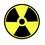# Determinants 3.5

This is when we investigate how we find the formulae for the determinants of matrixes of bigger size.

We remember that the formulas for the determinants are $$a_{11}a_{22}-a_{12}a_{21}$$ (for a 2 by 2 matrix) and $$a_{11}a_{22}a_{33}+a_{12}a_{23}a_{31}+a_{13}a_{21}a_{32}-a_{12}a_{21}a_{33}-a_{11}a_{23}a_{32}-a_{13}a_{22}a_{32}$$ (for a 3 by 3 matrix)

First, we take each entry as $a_{mn}$

FYI, I am writing this as a note as I do not know how to put it in questions.

1. Look at the first number of each entry. I mean the '123' in each $a_{11}a_{22}a_{33}$ We take out the first number (m) of each 'a'. They are all consecutive.

2. Now, we look at the second number (n). They all have numbers 1, 2, and 3 in total, but jumbled up in all combinations: 123, 132, 213, 231, 312, 321.

3. Now, we lastly have to see what sign follows which entry. We will look at the 'n'. 123, 231 and 312 have a positive sign to it. 321, 213 and 132 have a negative sign to it. We take each set of three numbers and look at how many times you have to swap one number with another to get back '123...' in the least number of swaps. If it is even, that entry has a positive sign. If it is odd, that entry has a negative sign. Here's some examples:

123 (0)

231-->132-->123(2)

312-->321-->123(2)

However,

321-->123(1)

213-->123(1)

132-->123(1)

With these three rules, we can determine the determinant for any square matrix.

From this note onwards, I will try to find patterns in some matrixes. So in the case you don't understand yet, you can try 4 (without guess1ng).Note by Aloysius Ng
6 years, 8 months ago

This discussion board is a place to discuss our Daily Challenges and the math and science related to those challenges. Explanations are more than just a solution — they should explain the steps and thinking strategies that you used to obtain the solution. Comments should further the discussion of math and science.

When posting on Brilliant:

• Use the emojis to react to an explanation, whether you're congratulating a job well done , or just really confused .
• Ask specific questions about the challenge or the steps in somebody's explanation. Well-posed questions can add a lot to the discussion, but posting "I don't understand!" doesn't help anyone.
• Try to contribute something new to the discussion, whether it is an extension, generalization or other idea related to the challenge.

MarkdownAppears as
*italics* or _italics_ italics
**bold** or __bold__ bold
- bulleted- list
• bulleted
• list
1. numbered2. list
1. numbered
2. list
Note: you must add a full line of space before and after lists for them to show up correctly
paragraph 1paragraph 2

paragraph 1

paragraph 2

[example link](https://brilliant.org)example link
> This is a quote
This is a quote
    # I indented these lines
# 4 spaces, and now they show
# up as a code block.

print "hello world"
# I indented these lines
# 4 spaces, and now they show
# up as a code block.

print "hello world"
MathAppears as
Remember to wrap math in $$ ... $$ or $ ... $ to ensure proper formatting.
2 \times 3 $2 \times 3$
2^{34} $2^{34}$
a_{i-1} $a_{i-1}$
\frac{2}{3} $\frac{2}{3}$
\sqrt{2} $\sqrt{2}$
\sum_{i=1}^3 $\sum_{i=1}^3$
\sin \theta $\sin \theta$
\boxed{123} $\boxed{123}$